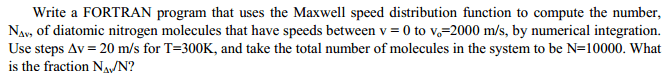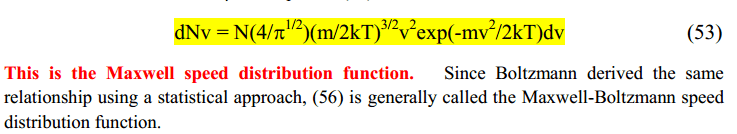# Basic Maxwell Speed Distribution Function

McAfee

## Homework Statement## Homework Equations## The Attempt at a Solution

I DO NOT NEED HELP WRITING THE PROGRAM! I'm just trying to figure out the basics behind it. Since this is an integral I will be solving this problem by using riemann sums. Where I'm having the most trouble is in the actually computation.

These are my constants:
N=100000
m=28.02
T=300
k=1.38*10^(-23)

For example I can look at the first case, v=0 and realize that I will get a result of 0.

Now when a substitute in v=20 and try to solve, my calculator gets an error that says INF.

Does anyone know what I'm doing wrong here.

Staff Emeritus